Question

# Qd=680-9Px-6I+4Py where Qd=quantity of good X demanded, Px=price of good X, I=Income, and Py=price of related...

Qd=680-9Px-6I+4Py

where Qd=quantity of good X demanded, Px=price of good X, I=Income, and Py=price of related good Y. From the demand function, it is apparent that good X is:

I. a normal good

II. an inferior good

III. a substitute for good Y

IV. a complement with good Y

a. II only

b. both I and III

c. both I and IV

d. both II and III

e. both II and IV

Ans.- (D)

It is an inferior good because dQ/dI = -6 <0

It is a substitute good because dQ/dPy>0

If you have any doubt feel free to ask.

#### Earn Coins

Coins can be redeemed for fabulous gifts.

Similar Homework Help Questions
• ### QD=8000-2P2 +0.4 I-2 PY +SP2 QD = Quantity demanded of good & PX = Price of...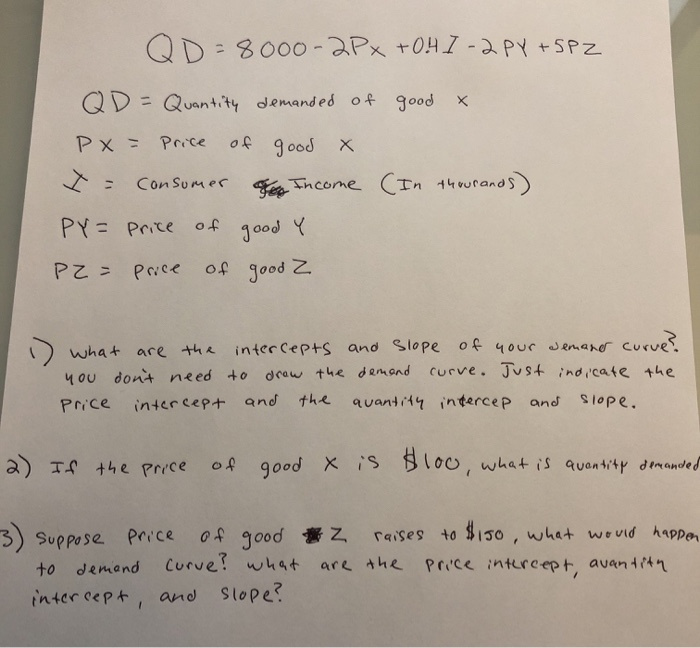QD=8000-2P2 +0.4 I-2 PY +SP2 QD = Quantity demanded of good & PX = Price of good x I= consumer of Income (In thousands) PY= Price of good Y P2= Price of good 2 1) what are the you don't need Price intercept intercepts and slope of your demaner curve? to draw the demand curve. Just indicate the and the quantity intercep and slope. 2) If the price of good x is \$100, what is quantity demanded 3) suppose price...

• ### The demand for good X is given by QXd = 6,000 - (1/2)PX - PY +...

The demand for good X is given by QXd = 6,000 - (1/2)PX - PY + 9PZ + (1/10)M Research shows that the prices of related goods are given by Py = \$6,500 and Pz = \$100, while the average income of individuals consuming this product is M = \$70,000. a. Indicate whether goods Y and Z are substitutes or complements for good X. Good Y is:  (Click to select)  a substitute  neither complement nor substitute  a complement  . Good Z is:  (Click to select)  a complement  a...

• ### 4. Given the demand function Q=98.6-2.3P+3.1P,-2.1Y where Q, is the quantity demanded, Px is the price...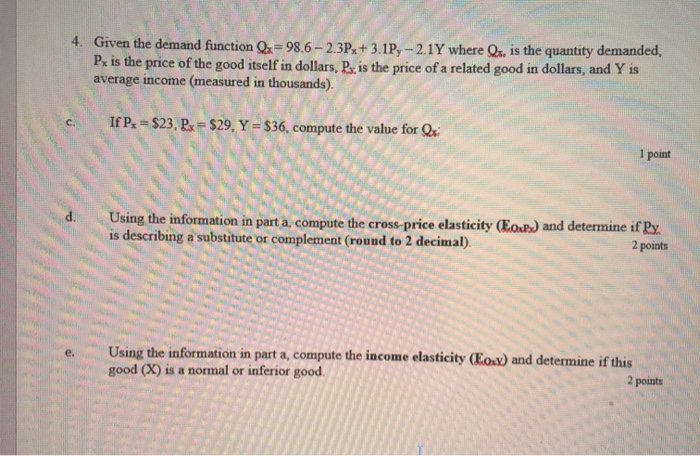4. Given the demand function Q=98.6-2.3P+3.1P,-2.1Y where Q, is the quantity demanded, Px is the price of the good itself in dollars, P is the price of a related good in dollars, and Y is average income (measured in thousands). If P \$23, Ps \$29, Y = \$36, compute the value for Q C 1 point Using the information in part a, compute the cross-price elasticity (Eo) and determine if Py is describing a substitute or complement (round to 2...

• ### Assume the demand function for good X can be written as Qd = 80 - 3Px...

Assume the demand function for good X can be written as Qd = 80 - 3Px + 4Py + 10I, where Px = the price of X, Py is the price of Y and I is consumer income. If the price of Y decreases by 2 dollars, what is the change in Px have to be in order to keep the quantity demanded of X unchanged by the change in the price of Y?

• ### Qp-8000-2Px+0.41-2Py+5Pz Where OD quantity demanded of good X Px price of good X Iconsumer income, in...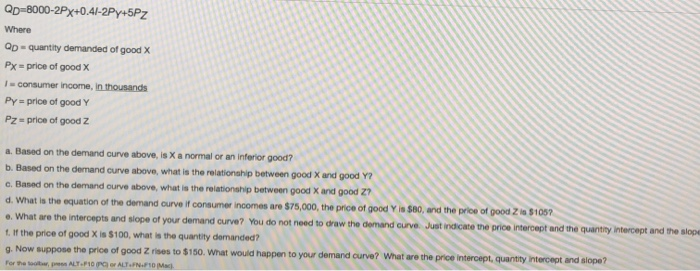Qp-8000-2Px+0.41-2Py+5Pz Where OD quantity demanded of good X Px price of good X Iconsumer income, in thousands Py price of good Y Pz price of good Z a. Based on the demand curve above, is X a normal or an inferior good? b. Based on the demand curve above, what is the relationahip between good X and good Y? c. Based on the demand ourve above, what is the relationship between good X and good Z7 d. What is the...

• ### Suppose Qxd = 10,000 - 2 Px + 3 Py - 4.5M, where Px = \$100,...

Suppose Qxd = 10,000 - 2 Px + 3 Py - 4.5M, where Px = \$100, Py = \$50, and M = \$2,000. (Note that Qdx is the quantity demanded of Good X, Px is the price of Good X, Py is the price of another product called Good Y, and M stands for income available.) Use this information to answer the following three parts of question 6. a. For this demand equation, what is the P intercept? b. For...

• ### The demand curve is given by: Qdx=500-1.5Px-0.2I-2Py+Pz Where Qdx= quantity demanded of good X Px= Price...

The demand curve is given by: Qdx=500-1.5Px-0.2I-2Py+Pz Where Qdx= quantity demanded of good X Px= Price of good X I= income (in thosands) Py= Price of good Y Pz= Price of good Z A. Is good X a normal or inferior good? Why? B. What is the relationship between goods X & Y? Why? C. What is the relationship between goods X & Z? Why? D. What is the equation of this demand curve if income is \$40,000, the price...

• ### Assume a demand equation for good'x: where pown price of the good Q-quantity demanded Py price...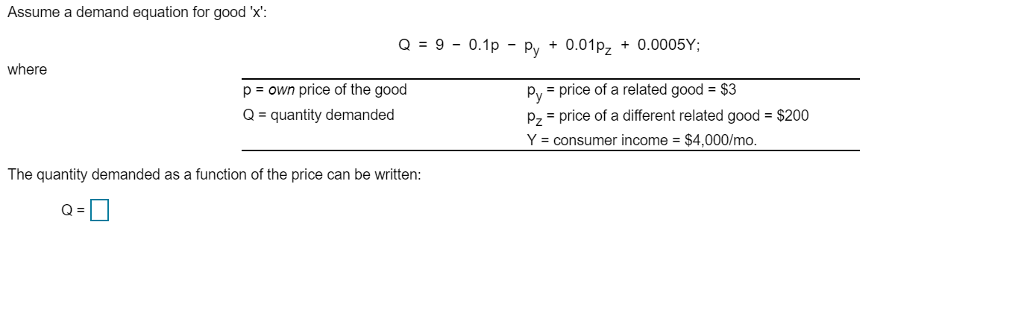Assume a demand equation for good'x: where pown price of the good Q-quantity demanded Py price of a related good \$3 Pz price of a different related good \$200 Y = consumer income = \$4,000/mo The quantity demanded as a function of the price can be written:

• ### Suppose the demand for good X is given by Xdx=20-Px+2Py+M. The price of good X is...

Suppose the demand for good X is given by Xdx=20-Px+2Py+M. The price of good X is \$5, the price of good Y is \$15, and the income is \$150. How much of good X will be purchased? Is good Y a substitute or complement of good X? Is good X a normal good or an inferior good?

• ### Consider the following demand function for good x -9-0.1p-Py+0.01p2+0.001Y, where Own price, P \$30 Quantity demanded...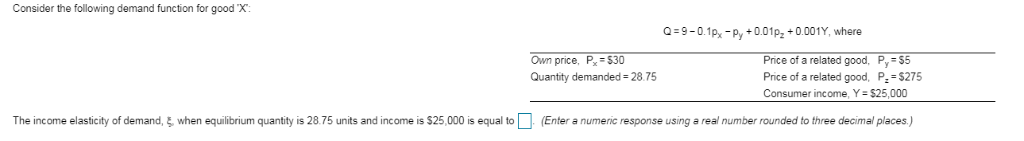Consider the following demand function for good x -9-0.1p-Py+0.01p2+0.001Y, where Own price, P \$30 Quantity demanded 28.75 Price of a related good, Py \$5 Price of a related good, P \$275 Consumer income, Y- \$25,000 The income elasticity of demand,when equilibrium quantity is 28.75 units and income is \$25,000 is equal to (Enter a numeric response using a real number rounded to three decimal places)